Examples

Chapter 7 Class 8 Comparing Quantities
Serial order wise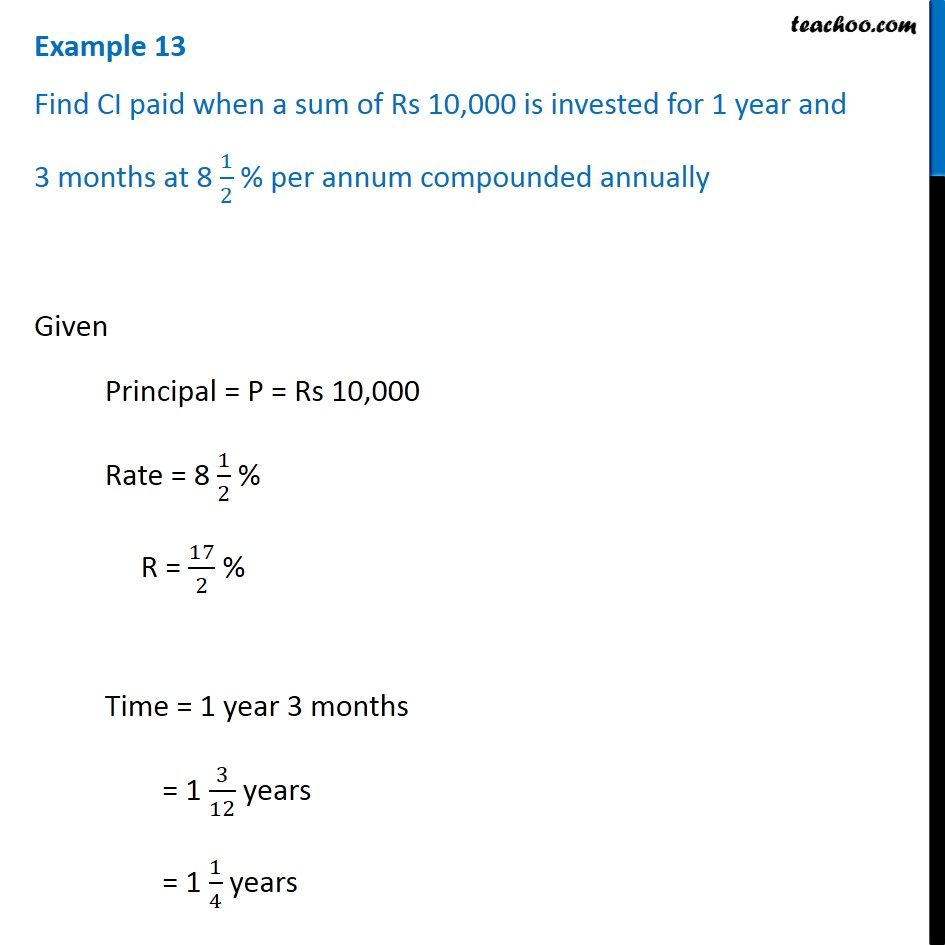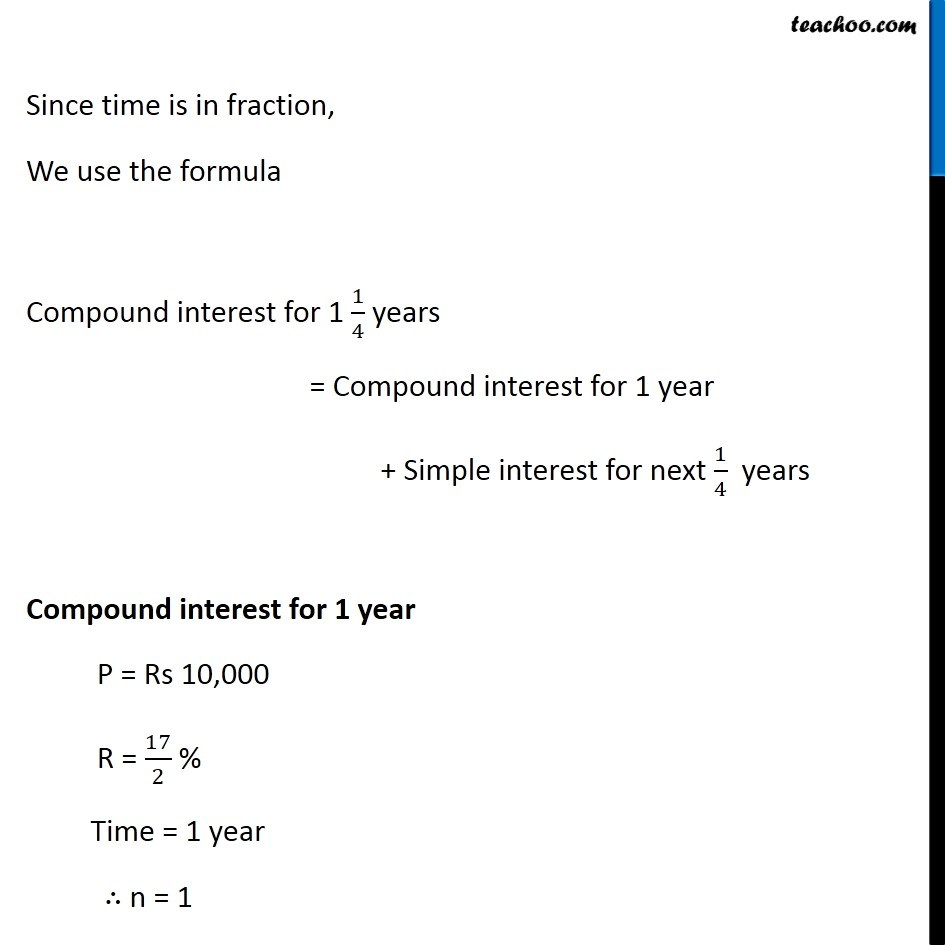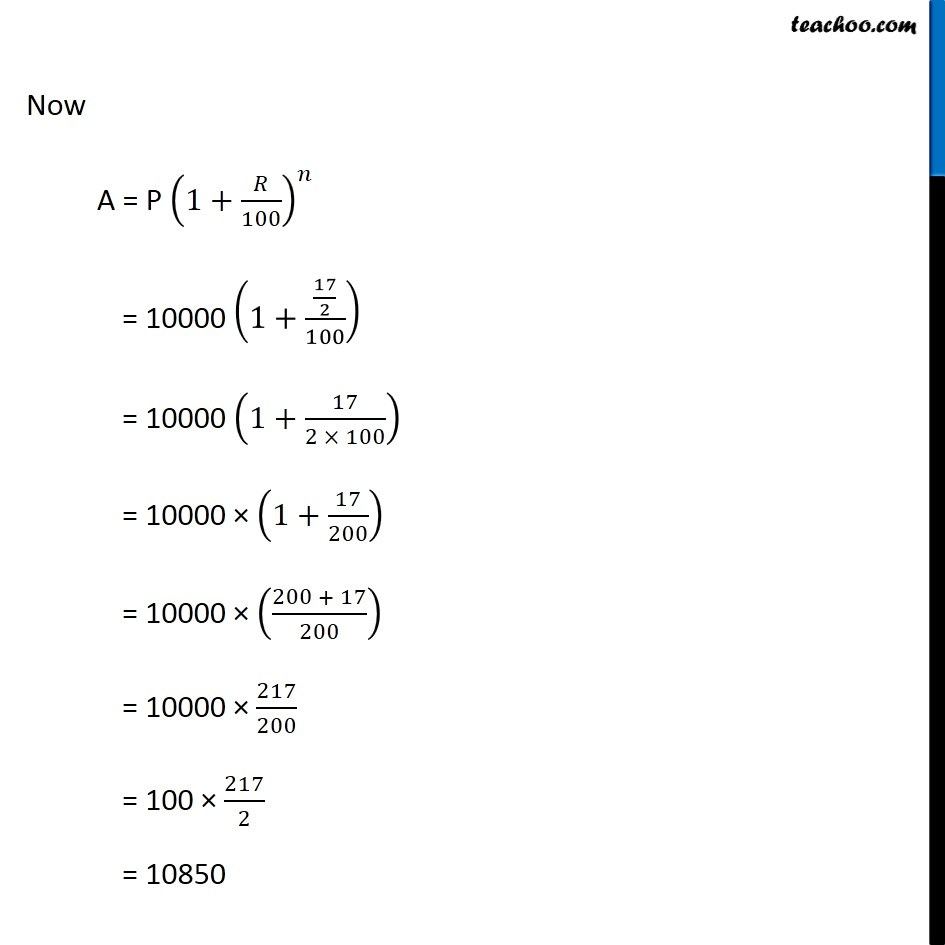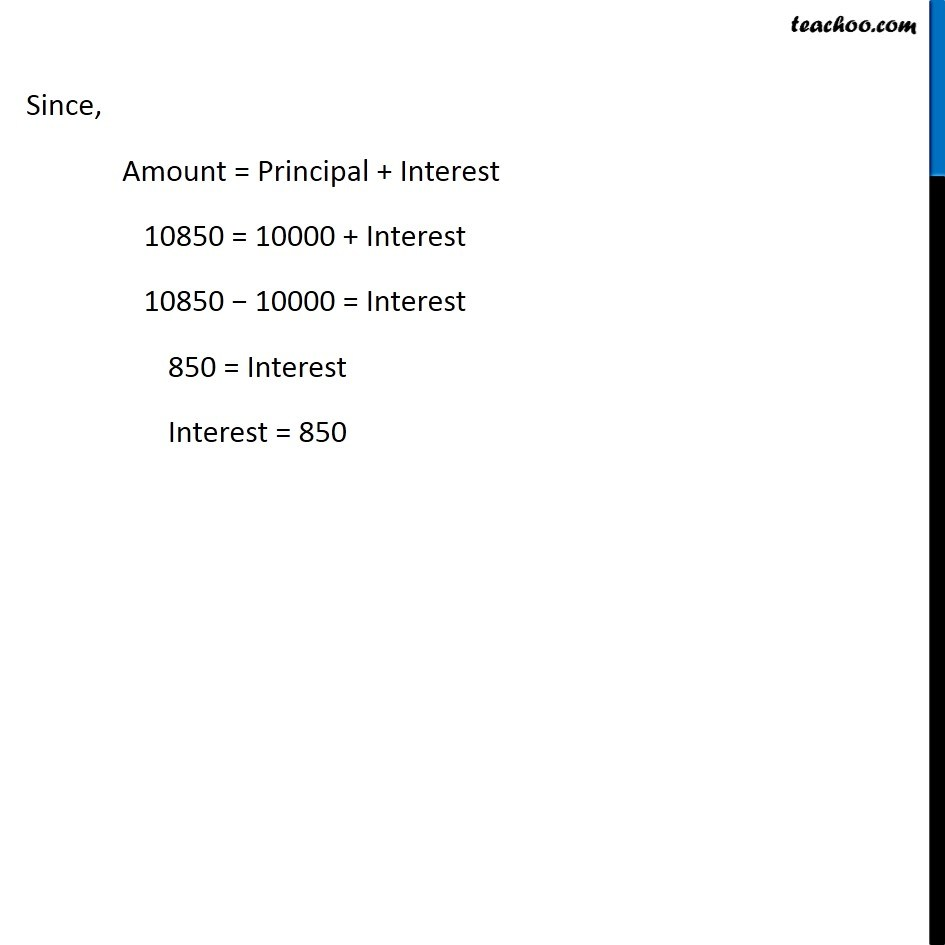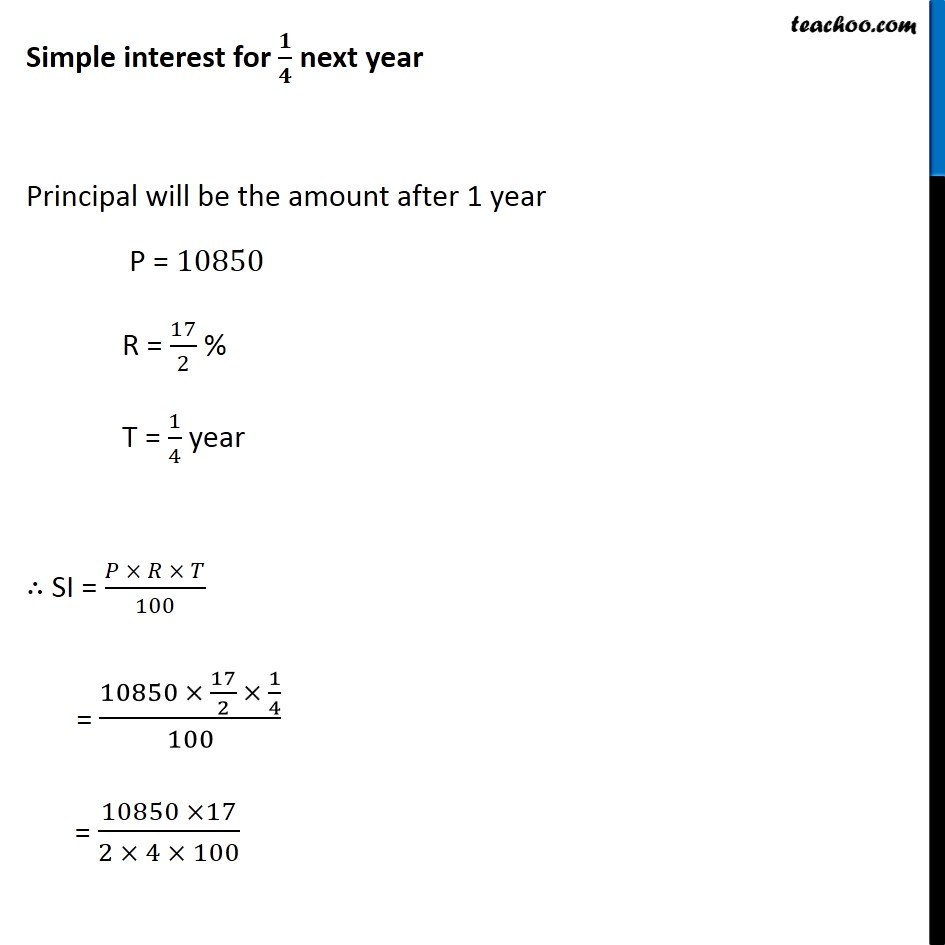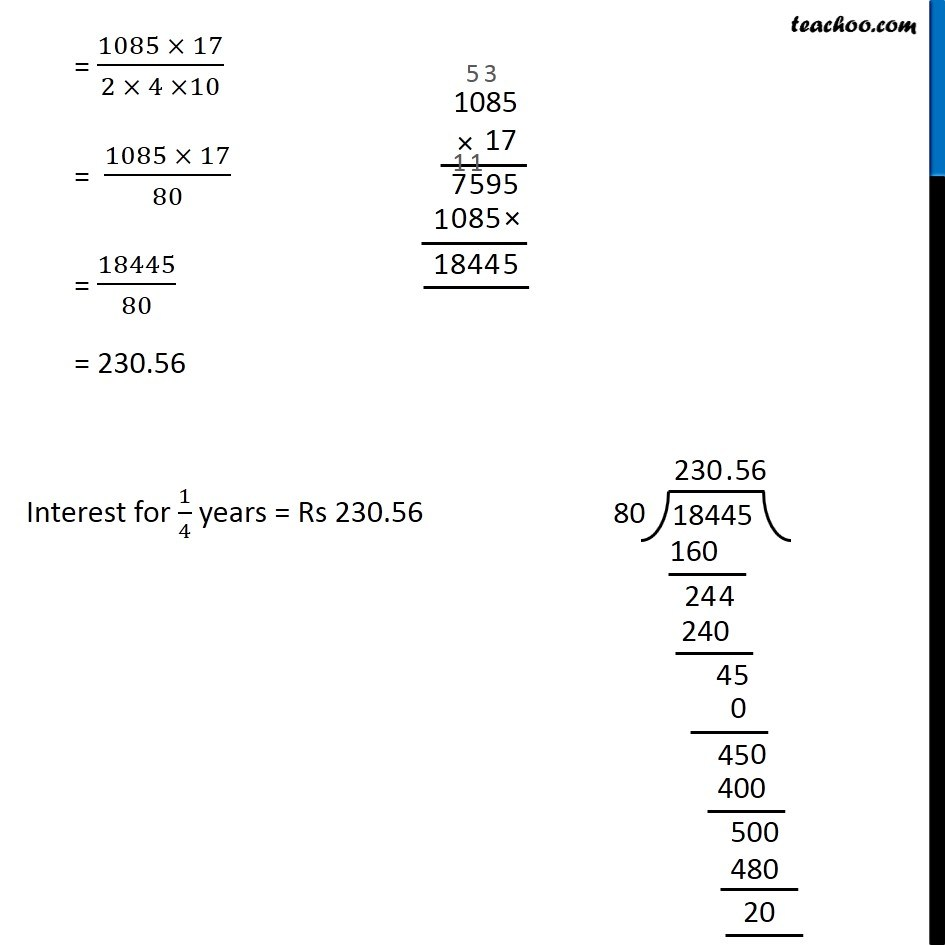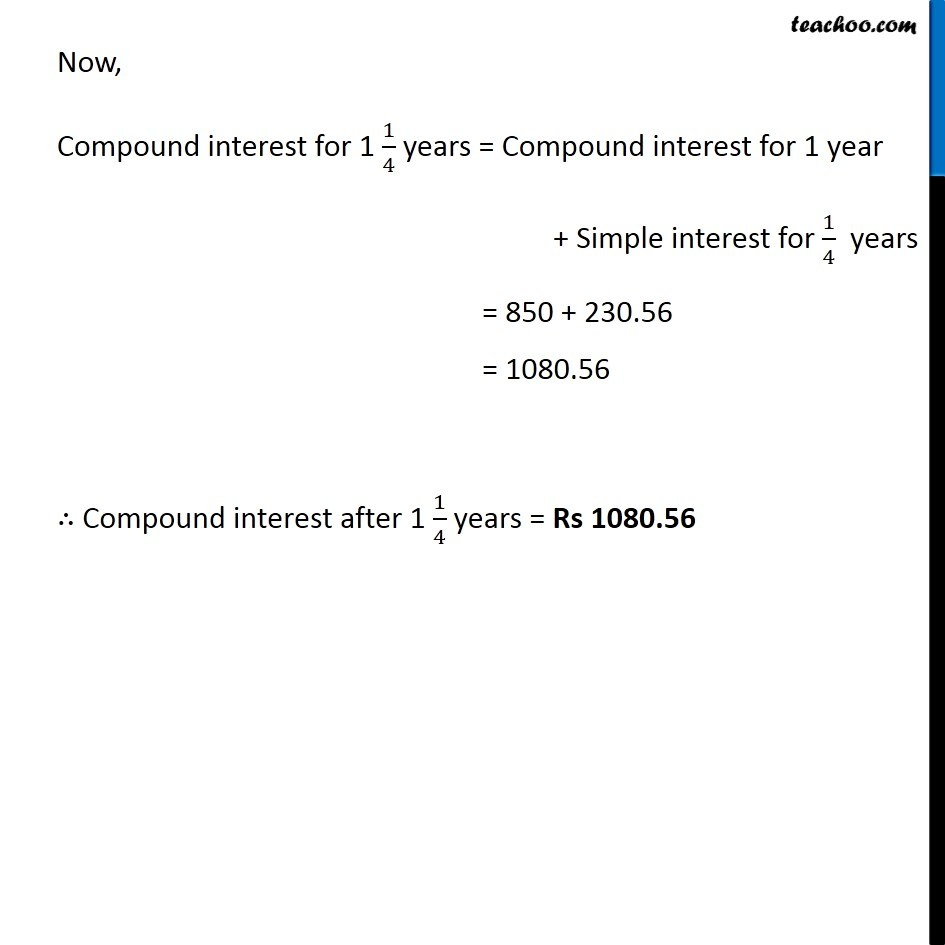Learn in your speed, with individual attention - Teachoo Maths 1-on-1 Class

### Transcript

Question 6 Find CI paid when a sum of Rs 10,000 is invested for 1 year and 3 months at 8 1/2 % per annum compounded annually Given Principal = P = Rs 10,000 Rate = 8 1/2 % R = 17/2 % Time = 1 year 3 months = 1 3/12 years = 1 1/4 years Since time is in fraction, We use the formula Compound interest for 1 1/4 years = Compound interest for 1 year + Simple interest for next 1/4 years Compound interest for 1 year P = Rs 10,000 R = 17/2 % Time = 1 year ∴ n = 1 Now A = P (1+𝑅/100)^𝑛 = 10000 (1+(17/2)/100) = 10000 (1+17/(2 × 100)) = 10000 × (1+17/200) = 10000 × ((200 + 17)/200) = 10000 × 217/200 = 100 × 217/2 = 10850 Since, Amount = Principal + Interest 10850 = 10000 + Interest 10850 − 10000 = Interest 850 = Interest Interest = 850 Simple interest for 𝟏/𝟒 next year Principal will be the amount after 1 year P = 10850 R = 17/2 % T = 1/4 year ∴ SI = (𝑃 × 𝑅 × 𝑇)/100 = (10850 × 17/2 × 1/4)/100 = (10850 ×17)/(2 × 4 × 100) = (1085 × 17)/(2 × 4 ×10) = (1085 × 17)/80 = 18445/80 = 230.56 Interest for 1/4 years = Rs 230.56 Now, Compound interest for 1 1/4 years = Compound interest for 1 year + Simple interest for 1/4 years = 850 + 230.56 = 1080.56 ∴ Compound interest after 1 1/4 years = Rs 1080.56